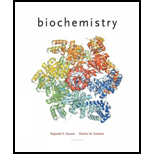# Answers to all problems are at the end of this book. Detailed solutions are available in the Student Solutions Manual, Study Guide, and Problems Book . Polyprotic Adds: Phosphate Species Abundance at Different pHs What are the approximate fractional concentrations of the following phosphate species at pH values of 0,2, 4, 6, 8, 10, and 12? H 3 PO 4 Η 2 ΡΟ 4 - HPO 4 2- PO 4 3-### Biochemistry

6th Edition
Reginald H. Garrett + 1 other
Publisher: Cengage Learning
ISBN: 9781305577206

#### Solutions

Chapter
Section### Biochemistry

6th Edition
Reginald H. Garrett + 1 other
Publisher: Cengage Learning
ISBN: 9781305577206
Chapter 2, Problem 8P
Textbook Problem
396 views

## Answers to all problems are at the end of this book. Detailed solutions are available in the Student Solutions Manual, Study Guide, and Problems Book.Polyprotic Adds: Phosphate Species Abundance at Different pHs What are the approximate fractional concentrations of the following phosphate species at pH values of 0,2, 4, 6, 8, 10, and 12? H3PO4 Η2ΡΟ4- HPO42- PO43-

Interpretation Introduction

(a)

To calculate:

The approximate fractional concentrations of H3PO4 at pH values of 0,2,4,6,8,10, and 12.

Concept Introduction:

Phosphoric acid has three H+ dissociations.

H3PO4H++H2PO4     pKa1=2.15H2PO4H++HPO42     pKa2=7.20HPO42H++PO43        pKa3=12.4

The Henderson-Hasselbalch equation is as follows:

pH=pKa+log[A][HA]

### Explanation of Solution

Rearranging the Henderson-Hasselbalch equation as follows:

pH=pKa+log[ A ][HA][ A ][HA]=antilog(pHpKa)

At particular pH, [H2PO4-][H3PO4] = x, [HPO42-][H2PO4-] = y, [PO43-][HPO42-] = z

Fraction of any species at a particular pH is concentration of the species divided by the sum of the concentration of all species.

fH3PO4=[H3PO4][H3PO4]+[H2PO4-]+[HPO42-]+[PO43-]

We can rewrite the above equation as a function of x, y, and z

Interpretation Introduction

(b)

To calculate:

The approximate fractional concentrations of H2PO4- at pH values of 0,2,4,6,8,10, and 12 should be calculated

Introduction:

Phosphoric acid has three H+ dissociations.

H3PO4H++H2PO4     pKa1=2.15H2PO4H++HPO42     pKa2=7.20HPO42H++PO43        pKa3=12.4

The Henderson-Hasselbalch equation is as follows:

pH=pKa+log[A][HA]

Interpretation Introduction

(c)

To calculate:

The approximate fractional concentrations of HPO42- at pH values of 0,2,4,6,8,10, and 12.

Introduction:

Phosphoric acid has three H+ dissociations.

H3PO4H++H2PO4     pKa1=2.15H2PO4H++HPO42     pKa2=7.20HPO42H++PO43        pKa3=12.4

The Henderson-Hasselbalch equation is as follows:

pH=pKa+log[A][HA]

Interpretation Introduction

(d)

To calculate:

The approximate fractional concentrations of PO43- at pH values of 0,2,4,6,8,10, and 12.

Concept Introduction:

Phosphoric acid has three H+ dissociations.

H3PO4H++H2PO4     pKa1=2.15H2PO4H++HPO42     pKa2=7.20HPO42H++PO43        pKa3=12.4

The Henderson-Hasselbalch equation is as follows:

pH=pKa+log[A][HA]

### Still sussing out bartleby?

Check out a sample textbook solution.

See a sample solution

#### The Solution to Your Study Problems

Bartleby provides explanations to thousands of textbook problems written by our experts, many with advanced degrees!

Get Started

Find more solutions based on key concepts
Vitamin D functions as a hormone to help maintain bone integrity. T F

Nutrition: Concepts and Controversies - Standalone book (MindTap Course List)

Lignin supports. the stems of ___ . a. mosses b. hornworts c. ferns d. liverworts

Biology: The Unity and Diversity of Life (MindTap Course List)

Twenty people participate in a tug-of-war. The two teams of ten people are so evenly matched that neither team ...

Physics for Scientists and Engineers, Technology Update (No access codes included)